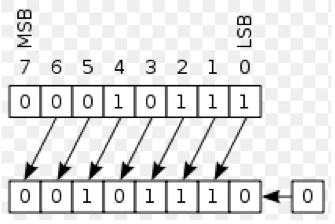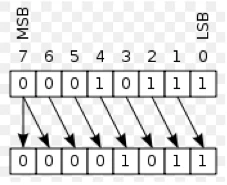Java provides a rich set of operators to manipulate variables. We can divide all the Java operators into the following groups:

• Arithmetic Operators
• Relational Operators
• Bitwise Operators
• Logical Operators
• Assignment Operators
• Misc Operators

# The Arithmetic Operators

Arithmetic operators are used in mathematical expressions in the same way that they are used in algebra. The following table lists the arithmetic operators:

Assume integer variable A holds 10 and variable B holds 20 then:

Operator Description Example
+ Addition - Adds values on either side of the operator A + B = 30
- Subtracts - Subtract right hand operand from left hand operand A - B = 10
* Multiplication - Multiplies values on either side of the operator A * B = 200
/ Division - Divides left hand operand by right hand operand B / A = 2
% Modulus - Divides left hand operand by right hand operand and return remainder B % A = 0
++ Increment - Increase value of operand by 1 B++ = 21
Decrement - Decrease value of operand by 1 B– = 19

# Pre and Post Increment/Decrement

Operators Description
x++ Post increment: add 1 to the value, The value is returned before the increment is made e.g x= 1, y = x++, then value of y = 1 & value of x = 2
x– Post decrement: subtract 1 from the value, The value is returned before the decrement is made e.g x= 1, y = x–, then value of y = 1 & value of x = 0
++x Pre increment: add 1 to the value, The value is returned after the increment is made e.g x= 1, y = ++x, then value of y = 2 & value of x = 2
–x Pre decrement: subtract 1 from the value, The value is returned after the decrement is made e.g x= 1, y = –x, then value of y = 0 & value of x = 0

# The Relational Operators

There are following relational operators supported by Java language. Whenever the relational operators are used, the output results to a Boolean value.

Assume variable A holds 10 and variable B holds 20 then:

Operator Description Example
== Checks if the value of two operands are equal, if yes the condition becomes true (A == B) is false
== Checks if the value of two operands are not equal, if yes the condition becomes true (A == B) is true
> Checks if the value of left operand is greater than right operand, if yes the condition becomes true (A > B) is false
< Checks if the value of left operand is lesser than right operand, if yes the condition becomes true (A < B) is true
>= Checks if the value of left operand is greater or equal to right operand, if yes the condition becomes true (A > B) is false
<= Checks if the value of left operand is less or equal to right operand, if yes the condition becomes true (A < B) is true

# The Bitwise Operators

Java defines several bitwise operators which can be applied to the integer types, long, int, short, char, and byte.

Bitwise operator works on bits and perform bit by bit operation. Assume if a = 60; and b = 13; Now in binary format they will be as follows:

``````a = 0011 1100
b = 0000 1101
-----------------
a&b = 0000 1000
a|b = 0011 1101
a^b = 0011 0001
~a = 1100 0011
``````

# Bitwise AND (&)

A bitwise AND takes two binary representations of equal length and performs the logical AND operation on each pair of corresponding bits. In each pair, the result is 1 if the first bit is 1 AND the second bit is 1. Otherwise, the result is 0.

Truth table:

A B Output
0 0 0
0 1 0
1 0 0
1 1 1

# Bitwise OR (|)

A bitwise OR takes two bit patterns of equal length, and produces another one of the same length by matching up corresponding bits (the first of each; the second of each; and so on) and performing the logical inclusive OR operation on each pair of corresponding bits. In each pair, the result is 1 if the first bit is 1 OR the second bit is 1 OR both bits are 1, and otherwise the result is 0.

Truth Table:

A B Output
0 0 0
0 1 1
1 0 1
1 1 1

# Bitwise XOR (^)

A bitwise exclusive or takes two bit patterns of equal length and performs the logical XOR operation on each pair of corresponding bits. The result in each position is 1 if the two bits are different, and 0 if they are the same.

Truth Table:

A B Output
0 0 0
0 1 1
1 0 1
1 1 0

# Bitwise NOT (~)

The bitwise NOT, or complement, is a unary operation that performs logical negation on each bit, forming the ones’ complement of the given binary value. Digits which were 0 become 1, and vice versa.

Truth Table:

Input Output
0 1
1 0

# Left Shift («)

In a left arithmetic shift, zeros are shifted in on the right. The leftmost digit was shifted past the end of the register, and a new 0 was shifted into the rightmost position.A left arithmetic shift by n is equivalent to multiplying by 2^n.

# Right Shift (»)

In a right arithmetic shift, the sign bit is shifted in on the left, thus preserving the sign of the operand. The rightmost 1 was shifted out (perhaps into the carry flag), and a new 0 was copied into the leftmost position, preserving the sign of the number.A right arithmetic shift by n of a two’s complement value is equivalent to dividing by 2n and rounding toward negative infinity.

The following table lists the bitwise operators, Assume integer variable A holds 60 and variable B holds 13 then:

Operator Description Example
& Binary AND Operator copies a bit to the result if it exists in both operand (A & B) will give 12 which is 0000 1100
| Binary OR Operator copies a bit if it exists in either operand (A | B) will give 61 which is 0011 1101
^ Binary XOR Operator copies a bit if it is set in one operand but not both (A ^ B) will give 49 which is 0011 0001
~ Binary ones complements Operator is Unary and has effect of ‘flipping’ bits (~A ) will give -60 which is 1100 0011
« Binary Left shift operator. The left operand value is moved left by number of bits specified by right operand A « 2 will give 240 which is 1111 0000
» Binary Right shift operator. The left operand value is moved right by number of bits specified by right operand A » 2 will give 15 which is 1111
»> Shift right zero fill operator. the left operands value is moved right by the number of bits specified by the right operand and shifted values are filled up with zeros A »> 2 will give 15 which is 0000 1111

``````public class Entry {
public static void main(String[] args) {
// create truth table for & (boolean logical AND) operator
System.out.printf( "%s\n%s: %b\n%s: %b\n%s: %b\n%s: %b\n\n",
"Boolean logical AND (&)", "false & false", ( false & false ),
"false & true", ( false & true ),
"true & false", ( true & false ),
"true & true", ( true & true ) );

// create truth table for | (boolean logical inclusive OR) operator
System.out.printf( "%s\n%s: %b\n%s: %b\n%s: %b\n%s: %b\n\n",
"Boolean logical inclusive OR (|)",
"false | false", ( false | false ),
"false | true", ( false | true ),
"true | false", ( true | false ),
"true | true", ( true | true ) );

// create truth table for ^ (boolean logical exclusive OR) operator
System.out.printf( "%s\n%s: %b\n%s: %b\n%s: %b\n%s: %b\n\n",
"Boolean logical exclusive OR (^)",
"false ^ false", ( false ^ false ),
"false ^ true", ( false ^ true ),
"true ^ false", ( true ^ false ),
"true ^ true", ( true ^ true ) );
}
}
``````
``````Boolean logical AND (&)
false & false: false
false & true: false
true & false: false
true & true: true

Boolean logical inclusive OR (|)
false | false: false
false | true: true
true | false: true
true | true: true

Boolean logical exclusive OR (^)
false ^ false: false
false ^ true: true
true ^ false: true
true ^ true: false
``````